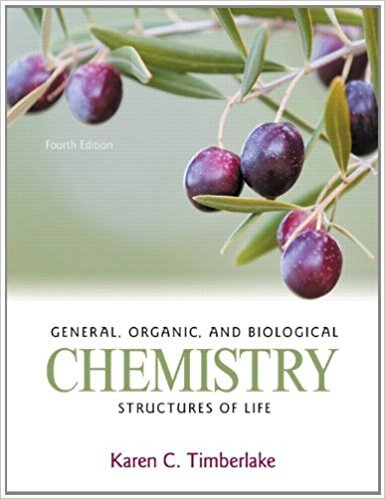# When the ore lead(II) sulfide burns in oxygen, the## Problem 57QP Chapter 6

General, Organic, and Biological Chemistry: Structures of Life | 4th Edition

• 2901 Step-by-step solutions solved by professors and subject experts
• Get 24/7 help from StudySoup virtual teaching assistantsGeneral, Organic, and Biological Chemistry: Structures of Life | 4th Edition

4 5 0 354 Reviews
14
1
Problem 57QP

When the ore lead(II) sulfide burns in oxygen, the products are solid lead(II) oxide and sulfur dioxide gas.a. Write the balanced equation for the reaction.b. How many grams of oxygen are required to react with 29.9 g of lead(II) sulfide?c. How many grams of sulfur dioxide can be produced when 65.0 g of lead(II) sulfide reacts?d. How many grams of lead(II) sulfide are used to produce 128 g of lead(II) oxide?

Step-by-Step Solution:

Solution 57 QPHere, we are calculating the each of the following.Step 1 of 4a.From the given,Lead (II) sulfide burns in the presence of Oxygen and to form lead oxide and sulphur dioxide.A chemical reaction is as follows.PbS(s) + O2(g) PbO(s) + SO2(g).Let’s balance the above chemical reaction Atoms Reactants ProductsBalanced/not balanced Pb 1 1Balanced S 1 1Balanced O 2 3Not balancedTo balance the equation, Subscript of O2 is 2 it is used as a coefficient for PbS ,PbO and SO2.And place the coefficient 3 in front of O2.2PbS(s) + 3O2(g) 2PbO(s) + 2SO2(g). Atoms Reactants ProductsBalanced/not balanced Pb 2 2Balanced S 2 2Balanced O 6 6 BalancedTherefore, the balanced chemical reaction in between lead(II) sulfide and oxygen is given below.2PbS(s) + 3O2(g) 2PbO(s) + 2SO2(g).____________________________________________________________________________Step 2 of 4b.Here, we are calculating the required grams of oxygen react with 29.9 lead(II) sulfide.Let’s calculate the molar mass of PbS and O2.Mass of PbS = 207 + 32.3 = 239.3Mass of O2 = 32.0 gWrite the equality and conversion factors between molar mass and grams.1 mole of PbS = 239.3 g of PbS.So, the conversion factors are and .1 mole of O2 = 32.0 g of Oxygen.So, the conversion factors are and Write the equality and conversion factors between mole and mole- factors of PbS and O2.2 moles of PbS = 3 mole of O2So, the conversion factors are and 29.9 g PbS = 6.00 g of Oxygen.Therefore, 6 g of Oxygen required to react with 29.9 lead(II) sulfide.______________________________________________________________________________

Step 3 of 4

Step 4 of 4

##### ISBN: 9780321750891

Unlock Textbook Solution

When the ore lead(II) sulfide burns in oxygen, the

×
Get Full Access to General, Organic, And Biological Chemistry: Structures Of Life - 4 Edition - Chapter 6 - Problem 57qp

Get Full Access to General, Organic, And Biological Chemistry: Structures Of Life - 4 Edition - Chapter 6 - Problem 57qp

I don't want to reset my password

Need help? Contact support

Need an Account? Is not associated with an account
We're here to help